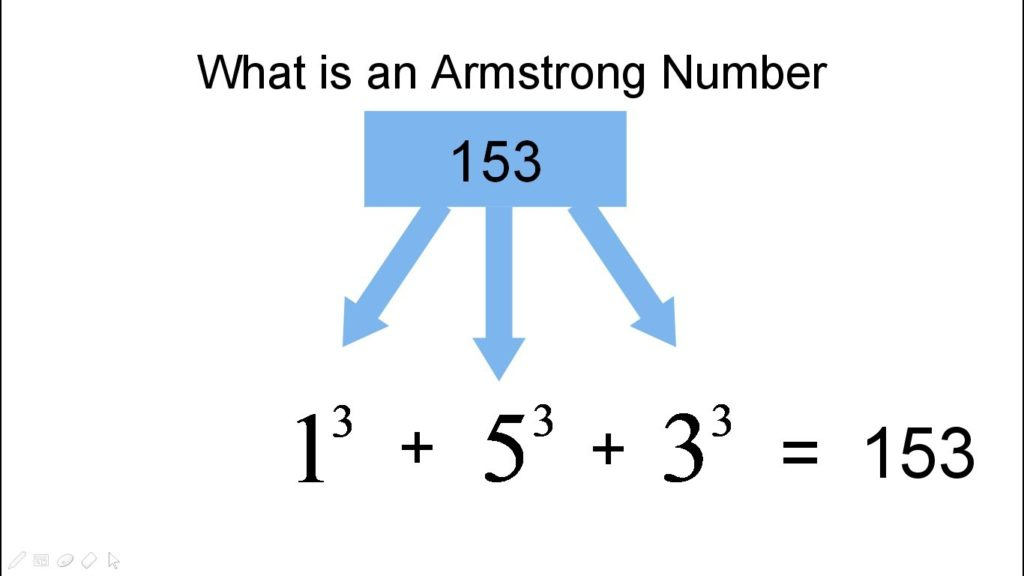# Armstrong Number In Java Program – 5 Simple Ways

Armstrong number in Java. Here we have written the code in four different ways standard, using for loop, recursion, while loop and also with different examples as like: between 100 and 999, between 1 to 1000 and between 1 to 500 with sample outputs and online execution tool embedded.

What is Armstrong Number?

A: An Armstrong number of three digits is an integer, where the sum of the cubes of its digits is equal to the number itself.

[table id=16 /]

Here is the example for you :

Consider the example: 371

A: 3^3 + 7^3 + 1^3 = 371 ( If you add those all numbers, the final digit should be same as given number ).

Here is the list of Java programs with different methods in different ways. Do check out the table of contents so that you will get an idea.1. Using static method Between 100 and 999

Here is the first sample program using the static method with sample output as well. Here we used the method ‘ Static  ‘ and taken numbers between 100 to 999. Once you are done with the execution, it automatically displays the Armstrong numbers between 100 and 999. Check out the sample output.

Output:

2. Using while Loop ( 1 to 500 )

There you go another method using while loop. Java, while loop is nothing but executing a set of statements repeatedly as long as condition, is true – here is the complete guide on while loop in Java with examples. In this case, we have taken the example of numbers from 1 to 500. Upon execution, you will get to know what are Armstrong numbers. Check out the output below so that you will get an idea.

Output:

3. Using Recursion ( Between 1 to 1000 )

Another method using recursion: A function that calls itself is called recursion. Here is the complete code with the sample program and the output of the program. Here we have taken the example of numbers from 1 to 1000. Check it out.

Output:

There you another method using bufferedreader. Do check it out.

Output:

5. Using for loop ( 100 to 500 )

Another sample program to print Armstrong number using for loop. Check out the program along with the sample output.

That’s it. If you have any doubts related to the above code. Just leave a comment here at the end of the post. We do try to help you out related to ant query asap.

More programs for you:

x

## Plus Star Pattern Java Program | Patterns

Java program to print plus star pattern program – We have written the below print/draw ...